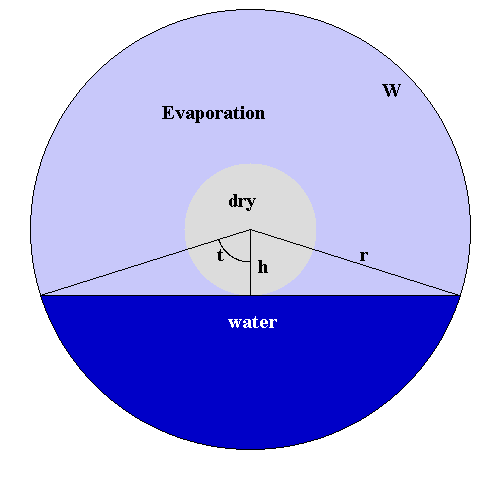A humidifier uses a vertical rotating disk of radius $r$ which is partially submerged in water. Evaporation is maximized if the exposed wetted area is maximized. The accompanying Figure shows the wetted area as light blue, the water as dark blue, and the dry area as light gray. If you have a black and white hard copy the light and dark blue may show as dark gray and black, respectively. You are looking along the axis of the disk, and it is rotating (clockwise or counterclockwise, it does not matter).
We want to know how to pick $h$, the distance from the center of the disk to the top of the water, so as to maximize the wetted area.
The submerged part of the disk forms a segment of a circle. If $t$ is the angle indicated in the Figure, i.e., half the angle defining the segment, then the area of the submerged part is
In terms of $t$ and $r$, the area $W$ of the wetted part is the area of the disk minus the area of the dry part minus the area of the submerged part, i.e.,
We now need to eliminate $h$ or $t$. Since we have and we can see similarly that Substituting these expressions in $W$ gives
$W =$ and $W' =$ . (Your answers will depend on $t$ and $r$.)
The equation $W'(t) = 0$ can be solved for $t$, giving
$t=$ radians which is degrees.

This means that where $\alpha =$ .
Check the solution after the set closes, for a more detailed discussion of these calculations.

You can earn partial credit on this problem.